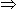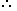# Simplification I

Q1
A man has Rs. 480 in the denominations of one-rupee notes, five-rupee notes and ten-rupee notes. The number of notes of each denomination is equal. What is the total number of notes that he has ?
Ans:

Let number of notes of each denomination be x.

Then x + 5x + 10x = 48016x = 480x = 30.

Hence, total number of notes = 3x = 90.

Q2

There are two examinations rooms A and B. If 10 students are sent from A to B, then the number of students in each room is the same. If 20 candidates are sent from B to A, then the number of students in A is double the number of students in B. The number of students in room A is?

Ans:

Let the number of students in rooms A and B be x and y respectively.

Then, x - 10 = y + 10x - y = 20 .... (i)

and x + 20 = 2(y - 20)x - 2y = -60 .... (ii)

Solving (i) and (ii) we get: x = 100 , y = 80.The required answer A = 100.

Q3

The price of 10 chairs is equal to that of 4 tables. The price of 15 chairs and 2 tables together is Rs. 4000. The total price of 12 chairs and 3 tables is?

Ans:

Let the cost of a chair and that of a table be Rs. x and Rs. y respectively.

Then, 10x = 4y   or   y =5x/2.15x + 2y = 400015x + 2 x 5x/2=400020x = 4000x = 200.

So, y = 5 x 200 / 2 = 500.

Hence, the cost of 12 chairs and 3 tables = 12x + 3y

= Rs. (2400 + 1500)

= Rs. 3900.

Q4

If a - b = 3 and a2 + b2 = 29, find the value of ab?

Ans:

(a - b)2 = a2 -2ab + b2ab = (a2 + b2) - (a - b)2

= 29 - 9 = 20ab = 10.

Q5

The price of 2 sarees and 4 shirts is Rs. 1600. With the same money one can buy 1 saree and 6 shirts. If one wants to buy 12 shirts, how much shall he have to pay ?

Ans:

Let the price of a saree and a shirt be Rs. x and Rs. y respectively.

Then, 2x + 4y = 1600 .... (i)

and x + 6y = 1600 .... (ii)

Divide equation (i) by 2, we get the below equation.

=> x + 2y = 800. --- (iii)

Now subtract (iii) from (ii)

x + 6y = 1600 (-)

x + 2y = 800

----------------

4y = 800

----------------

Therefore, y = 200.

Now apply value of y in (iii)

=> x + 2 x 200 = 800

=> x + 400 = 800

Therefore x = 400

Solving (i) and (ii) we get x = 400, y = 200.Cost of 12 shirts = Rs. (12 x 200) = Rs. 2400.